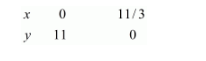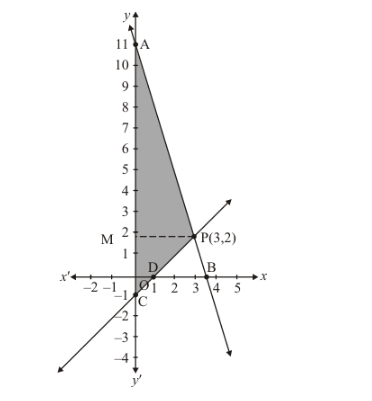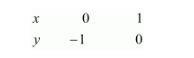# Solve the following system of linear equations graphically :`
Question:

Solve the following system of linear equations graphically :

3x + y − 11 = 0, − y − 1 = 0.
Shade the region bounded by these lines and y-axis. Also, find the area of the region bounded by the these lines and y-axis.

Solution:

The given equations are

$3 x+y-11=0$$\ldots \ldots( i ) x-y-1=0$$\ldots \ldots($ ii $)$

Putting $x=0$ in equation $(i)$, we get:

$\Rightarrow 3 \times 0+y=11$

$\Rightarrow y=11$

$x=0, \quad y=11$

Putting $y=0$ in equation $(i,$, we get:

$\Rightarrow 3 x+0=11$

$\Rightarrow x=11 / 3$

$x=11 / 3, \quad y=0$

Use the following table to draw the graph.Draw the graph by plotting the two points from table.$x-y=1$ ..(ii)

Putting $x=0$ in equation $(i i)$ we get:

$\Rightarrow 0-y=1$

$\Rightarrow y=-1$

$x=0, \quad y=-1$

Putting $y=0$ in equation $(i i)$, we get:

$\Rightarrow x-0=1$

$\Rightarrow x=1$

$x=1, \quad y=0$

Use the following table to draw the graph.Draw the graph by plotting the two points from table.

The two lines intersect at $P(3,2)$.

Hence $x=3$, $y=2$ is the solution of the given equations

The area enclosed by the lines represented by the given equations and the y−axis is shaded region in the figure

Now, Required area = Area of shaded region

$\Rightarrow$ Required area $=$ Area of PAC

$\Rightarrow$ Required area $=1 / 2($ base $\times$ height $)$

$\Rightarrow$ Required area $=1 / 2(A C \times P M)$

$\Rightarrow$ Required area $=1 / 2(12 \times 3)$ sq.units

Hence the required area is 18 sq. unit